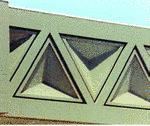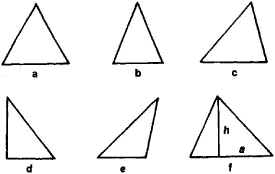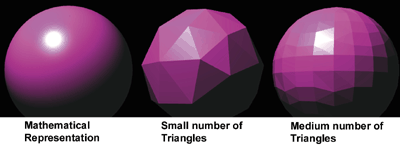# triangle

(redirected from digastric triangle)
Also found in: Dictionary, Thesaurus, Medical, Financial.

## triangle,

in mathematics, plane figure bounded by three straight lines, the sides, which intersect at three points called the vertices. Any one of the sides may be considered the base of the triangle. The perpendicular distance from a base to the opposite vertex is called an altitude. The area of a triangle is equal to one half the product of the base and the corresponding altitude. The line segment joining the midpoint of a side to the opposite vertex is called a median. All three altitudes of a triangle go through a single point, and all three medians go through a single (usually different) point. In Euclidean geometry the sum of the angles of a triangle is equal to two right angles (180°). If all three angles of a triangle are equal, the triangle is called equilateral. An isosceles triangle has two equal angles. A scalene triangle is one in which all three angles are different. A right triangle has one right angle. In geometry it is shown that two triangles are congruent (i.e., are the same shape and size) if, in general, any three independent parts (sides or angles) of one are the same as the corresponding three parts of the other. The rules of congruency make it possible, in trigonometry, to compute the sides and the angles of a triangle when three of these values are known. The triangle is the simplest of the polygons (i.e., it has the least possible number of sides). Since any polygon can be broken up into triangles by drawing various diagonals, a complete theory of the measurement of triangles provides a complete theory of the measurement of all polygons. In non-Euclidean geometries, the angles of a triangle are either less than two right angles (hyperbolic geometry) or more than two right angles (elliptic geometry).

## triangle,

in music, percussion instrument consisting of a steel rod bent into a triangle, open at one angle, and struck with a steel rod. Only since the end of the 18th cent. has it been an orchestral instrument, although it appeared in Europe much earlier. Its tinkling sound is of indefinite pitch and therefore blends with whatever harmonies the orchestra produces.

## Triangle

A plane geometrical figure with three sides and three angles; the equilateral triangle has both equal sides and equal angles.## Triangle

(religion, spiritualism, and occult)

Equivalent to the number three, the triangle concerns the Trinity. The Trinity of ancient Egypt included Isis, Osiris, and Horus; the Hindu Trinity, or Trimurti, includes Brahma, Vishnu, and Shiva; the Greek Trinity included Artemis, Selene, and Hecate. It is the symbol for the Triple Goddess: Maiden-Mother-Crone.

In its normal position, with the point at the top, the triangle is a symbol for fire; when inverted—with the point at the bottom—it is a symbol for water. Inverted and with the tip cut off, it is a symbol for earth; upright and with the tip cut off, it symbolizes air.

Wiccan traditions that work with a degree system use the inverted triangle as a symbol for the First Degree. Apex upward, it is placed above a pentagram as the sign of the Third Degree. The Greek letter D, or delta, is a triangle and is described as "the letter of the vulva" and as the "Holy Door." Barbara Walker points out that the Jewish tradition of triangular hamantaschen for Purim is an apparent adoption from the ancient Egyptian custom of making triangular cakes for public rituals.

Walking under a ladder is considered unlucky because a ladder leaning against a wall forms a triangle. The action breaks that triangle, thus breaking the sacred three and the Trinity.

In numerology the number three, the number of the triangle, is ruled by Jupiter and is attuned to the investigator, the scientist, and the seeker; it is also associated with the letters C, L, and U.

## Triangle

a part of the plane bounded by three line segments each pair of which has a common end point. The line segments are called the sides of the triangle, and the end points are called the vertices of the triangle. In English the term “triangle” is also applied to the figure formed by the three line segments.Figure 1

An equilateral triangle is a triangle with all three sides equal (Figure 1,a). In an isosceles triangle only two of the sides are equal (Figure 1,b). A triangle whose interior angles are all acute is called an acute triangle (Figure 1,c). If one of the angles is a right angle, the triangle is said to be a right triangle (Figure 1,d). If one of the angles is obtuse, the triangle is called an obtuse triangle (Figure 1,e).

A triangle cannot have more than one right angle or one obtuse angle, since the sum of all three angles is equal to the sum of two right angles, which is 180° or, in radians, π. The area of a triangle is ah/2, where a is the length of one of its sides, which is designated as the base, and h is the corresponding altitude of the triangle (Figure 1,f).

The length of each side of a triangle is smaller than the sum of the lengths of the other two sides and is greater than the difference between the lengths of the other two sides. Each of the following is a necessary and sufficient condition for two triangles to be congruent: (1) each side of one triangle is equal to the corresponding side of the other triangle; (2) two sides and the angle between them of one triangle are equal, respectively, to the corresponding sides and angle of the other triangle; and (3) two angles and the side between their vertices of one triangle are equal, respectively, to the corresponding angles and side of the other triangle. The numerical relations between the angles and sides of triangles are studied in trigonometry.

Triangles on a sphere are discussed in SPHERICAL GEOMETRY and SPHERICAL TRIGONOMETRY.

## Triangle

in mechanical drawing, an instrument for drawing angles. Triangles may be made of wood, plastic, or, less frequently, of metal. The most common triangles have angles of 30°, 60°, and 90° or 45°, 45°, and 90°. Sometimes one side of a triangle is graduated in, for example, millimeters and centimeters so that it can be used as a measuring scale.

## Triangle

a percussion instrument consisting of a steel bar bent in a triangular form but open at one angle. The triangle is suspended from a strap or string; when struck with a small metal rod, it produces a clear, ringing sound. The triangle is used in or chestras and other instrumental ensembles.

## triangle

[′trī‚aŋ·gəl]
(mathematics)
The figure realized by connecting three noncollinear points by line segments.

[′trī‚aŋ·gəl]
(astronomy)

## triangle

1. Geometry a three-sided polygon that can be classified by angle, as in an acute triangle, or by side, as in an equilateral triangle. Sum of interior angles: 180°; area: ½ base × height
2. Music a percussion instrument consisting of a sonorous metal bar bent into a triangular shape, beaten with a metal stick

## triangle

A three-sided polygon. In 3D graphics, the surfaces of 3D objects are broken down into triangles. Small numbers of triangles are used for flat surfaces, while large numbers are used to mold curved surfaces similar to the way a geodesic dome is constructed. The three points of every triangle (vertices) are computed on an X-Y-Z scale and must be recomputed each time the object is moved. See triangle setup and geometry calculations.

TrianglesThe smaller the triangle, the more realistic the curve. It takes an enormous number of triangles to simulate totally round objects. (Image courtesy of Intergraph Computer Systems.)
Site: Follow: Share:
Open / Close